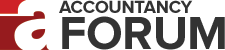computation of IRR - Printable Version +- Accountancy Forum (https://www.accountancy.com.pk/forum) +-- Forum: The Profession (https://www.accountancy.com.pk/forum/forumdisplay.php?fid=4) +--- Forum: Accounting and Audit (https://www.accountancy.com.pk/forum/forumdisplay.php?fid=7) +--- Thread: computation of IRR (/showthread.php?tid=8196) computation of IRR - Abuzar Qazi - 06-19-2010 Please guide me regarding the computation of iRR - huma_ahmed - 06-24-2010 IRR calculation involves the same equation that we used for the calculation of NPV.[)] The only difference is that while calculating IRR we would set the value of NPV equal to zero and then solve the equation for the value if âiâ. In other words, the value of âiâ, at which the net present value of the project equals zero would be considered as the internal rate of return of the project. NPV= -IO +CF1/ (1+IRR) + CF2/ (1+IRR) 2 = 0= -200,000 + 120,000/ (1+0.1) + 240,000/ (1+0.1)2 - imdad1 - 07-07-2010 Abuzar saeen how r u? well dear IRR is computed by the follwing steps 1)plot cash flows year wise 2)discount cash flow by any rate "x%" eg 10% 3) if present value is posative when discounted by X% above we will chose a rate higher than X% lets say 15% in order to bring the present value discounted at new rate NEGATIVE 4) now we have two rates and two values (one posative and one negative) now put in the following formula to get IRR IRR=a+{p/(p-n)}*(b-a) a=lower rate b=higher rate p=posative value (value we got using lower rate) n=negative value(value we got using higher rate) abuzar hope your query solved ) Huma do u agree with my response ??
quote
Originally posted by Abuzar Qazi
Please guide me regarding the computation of iRR
- Abuzar Qazi - 07-10-2010 Imdad AOA.........It means the next rate should bring down the negative PV....means k agr 15% pe bhy negative me nahi ati PV so we have to select another rate that should result in neagtive PV.. - imdad1 - 07-11-2010 Absolutely !!! ur right!!! this process is called interpolation however we can also compute irr by using two negative values by the same formula this process is called Extrapolation but their are issues with extrapolation regarding accuracy and correctnesss thus we always do interpolation
quote
Originally posted by Abuzar Qazi
Imdad AOA.........It means the next rate should bring down the negative PV....means k agr 15% pe bhy negative me nahi ati PV so we have to select another rate that should result in neagtive PV..
- IFRSbox.com - 07-12-2010
quote
Originally posted by Abuzar Qazi
Please guide me regarding the computation of iRR
Dear Abuzar, I just add to the previous comments. Why not trying to calculate it with simple excel formula? Simple steps 1. Put your cash flows in the table year by year, or period by period (careful - periods between individual rows should be the same, not irregular). 2. Use formula =IRR(here you enter the range of cells where your cash flows are, e.g. A1A13). The result is your internal rate of return. Best regards Silvia M./www.ifrsbox.com - yasir_live - 08-13-2010 KamranACA ki bharpoor kaamyabi k baad Abb ICAP pesh karta hay aap ki khidmat main KawishACA. Regards. - WARDAH - 08-14-2010 hahahahaha good one yasir